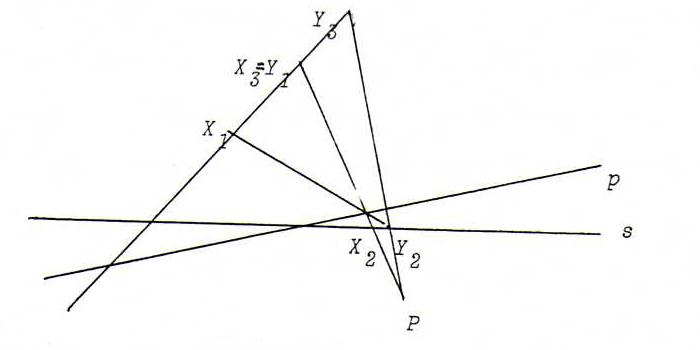### COURSE OF PROJECTIVE GEOMETRY

§ 14:

O55 Suppose A = ((a,b),(c,d)) meets the requirements. A maps (4,-5) to ρ(1,2) and (1,2) to σ(4,-5). We get A = μ((2.1),(-1,-2)).

O56 Suppose A = ((a,b),(c,d)) meets the requirements. Using A2 = λE, we find a2 = d2 and c(a+d) = b(a+d) = 0.
If a=d≠0 then b=c=0, but then the projectivity is trivial. So a=-d.
We find the eigenvalues by equating the characteristic polynomial λ2 - a2 - bc to 0. So hyperbolic if a2 + bc is positive, elliptic if a2 + bc is negative. If a2 + bc = 0, then the matrix is singular.

O57 Rename X3=Y1 and prove: Y3=X1P on p.(Y3=X1PY3=PX1Y2X1=PX1)

"→" : If Y3=X1 then Y2X1=PX1 and Y2X1.Y1X2 = PX1.PX2 = P. So P on p.

"→" : If P on p then P = p.Y1X2 so P op Y2X1. Then Y2X1=PX1 so Y3=X1.

O58 From the data it follows that (P, Q; A, A') = (P, Q; B, B') = -1 = (Q, P; B, B').
Hence, the projectivity that maps P to Q, and Q to P, and A to B, maps A' to B'.
(It is an involution because it interchanges P and Q.)